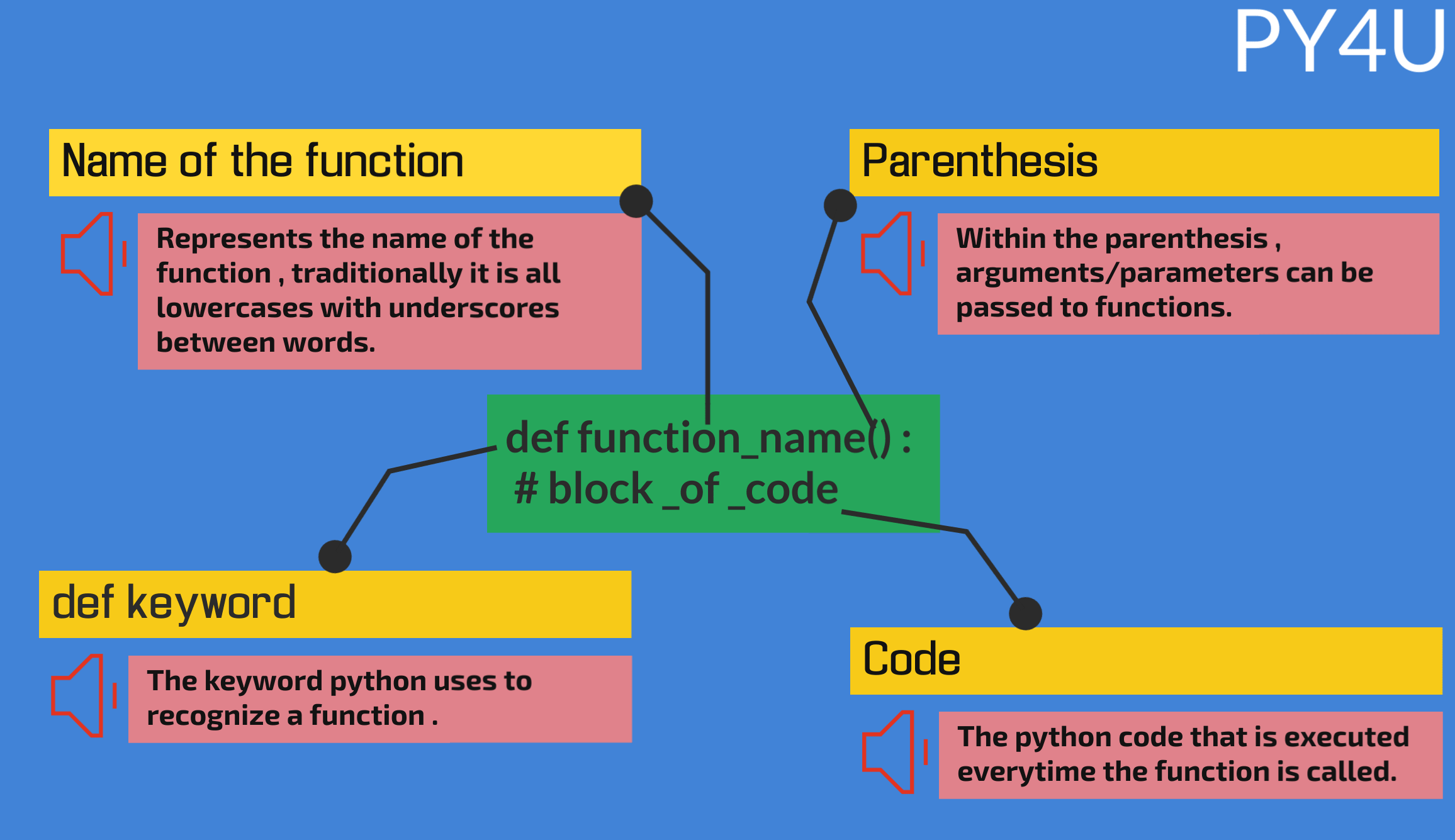Intro To Functions

Till now we have seen that in order to use a block of code in different sections of our programme , we'll need to basically re-write the whole code . This is where the role of a function comes into play . Functions are basically a block of code that performs some operation and returns the result wherever it is called. So instead of writing a particular block of code multiple times , simply using a function is a much better alternative.

The components of a function are explained below.Let us take a simple example where our function prints "hello world". To call a function ,remember to write the function name along with parenthesis.

def py4u():
print("hello world")

py4u()  # calling a function
output:
hello worldprint() is rarely used in a function , instead of print() , keyword return is a preferred choice.When dealing with functions , it is very rare that a function just prints a statement. Most of the times , functions will perform some sort of operation and return a value that can be stored in a variable so that it can be easily referenced later.Results of a print statement cannot be stored in a variable while return allows us to store the results of a function and therefore is preferred most of the times.

Let us take an example where we use the return statement in a function.

def py4u():
return 20

my_var = py4u()
print(3*my_var)
output:
60
passing arguments to a function

Most of the times , some arguments will be passed to the function and the function will use these parameters to perform some operation. Let us see this with help of an example.

In the example given below , the function is given a name and it returns a concatinated statement - 'hello! ' + name .

def py4u(name):
return 'hello! '+name

print(py4u('jack'))
output:
hello! jack

Let us take an example where we pass in 2 numbers to a function and it returns its product.

def py4u(num1,num2):
return num1 * num2

print(py4u(10,20))
output:
200

A parameter can also be assigned a default value , meaning when no value is passed to this parameter , its default value is used.In the code below , i have set the the value of num2 = 20 .

def py4u(num1,num2=20):
return num1 * num2

print(py4u(20))
output:
400Note that in the above example , if both the parameters are passed then the value of num2 will be overwritten to the newly passed value.While dealing with two parameters , it is recommended to not set default value for first parameter if you intend to pass only one argument. Python will throw an error because the value passed in function will overwrite the value of first parameter and second parameter will get no value. This will become more clear through the example given below.
def py4u(num1=20,num2):
return num1 * num2

print(py4u(20))

Popular Tutorials